# Turbulent Mixed Convection in Enclosure Containing a Circular/Square Heat Source

Turbulent Mixed Convection in Enclosure Containing a Circular/Square Heat Source

Said BouabdallahDjalloul Chati Badia Ghernaout Aissa Atia Aissa Laouirate

LME, Laboratory of Mechanics, University of Laghouat, Laghouat 03000, Algeria

Corresponding Author Email:
fibonsaid@gmail.com
Page:
446-454
|
DOI:
https://doi.org/10.18280/ijht.340314
|
Accepted:
|
Published:
30 September 2016
| Citation

OPEN ACCESS

Abstract:

In this study, we are interested to the turbulent mixed convection in a ventilated enclosure. A heat source is placed at the middle of the enclosure that’s equipped with two openings; one is located at the lower left corner and the other located in the upper right corner. The width of the opening "h" represents 1/5 of the side of the cavity. The walls of the cavity are maintained adiabatic. The temperature of the heat source Th is higher than the room temperature. The width of the heat source "D" is equal to the opening width "h" (D = h). The governing equations of turbulent mixed flow convection in the cavity are solved using finite volumes method. A model k-ε of two-equation was used for closure the equations of turbulent regimes. The Grashof number is set constant (Gr = 109) and the Reynolds number (Re) varied such that the Richardson number (Ri) takes the values ​​: 0.01, 0.05, 0.1, 1, 2, 5, 10, 20 and 30 (Ri = Gr / Re2). The effects of flow thermal, dynamic parameters, the geometry and heat source dimensions are presented and discussed.

Keywords:

Mixed convection, Ventilated enclosure, Heat source, k- ε standard turbulence model.

1. Introduction

The purpose of this work is to study the turbulent mixed convection in enclosure containing a heat source at the middle. The effect of the size of the source (D = 0.5h, h, 2h, 3h and 5h) and the shape of the heat source (square or circular) were examined. The effect of varying the size of the openings was also considered (h = L/10, L/4, L/3) in this study.

2. Problem Statement and Mathematical Formulation

A side of the enclosure having two small openings are considered, one located at the lower left corner and the other at the upper right corner. The two openings have the same width “h” which is equal to L/5 (L is the length of the adiabatic enclosure). The heat source (square or circular) is placed at the middle of the enclosure and have the dimension/diameter equal D. In this work we will examine the case of the turbulent mixed convection with a heat source (Fig.1).  So the following assumptions have been made: the fluid is incompressible and Newtonian, the Boussinesq approximation is valid, the two dimesionel flow is considred and the k-ε model of two-equation was used for closure the equations in turbulent regimes.  By introducing the following dimensionless variables and parameters, $X, Y=\frac{x, y}{D}, U, V=\frac{u, v}{v_{i n}}, P=\frac{p-p_{0}}{\rho v_{i n}^{2}}, K=\frac{k}{v_{i n}^{2}}, T=\frac{t-t_{0}}{t_{p}-t_{0}}, \varepsilon^{*}=\frac{\varepsilon}{v\left(V_{i n / L}\right)^{2}}$,$v^{*}=v_{t} / v$ and $H=D / L$ respectively for the dimensionless coordinate, velocity components, pressure, turbulent kinetic energy, temperature dissipation of turbulent kinetic energy, kinematic viscosity and dimensionless ratio.

## figure_1.pngFigure 1. Physical problem with boundary conditions

The dimensionless governing equations take the following forms:

Continuity equation:

$\frac{\partial U}{\partial X}+\frac{\partial V}{\partial Y}=0$(1)

Momentum equation in x-direction

$\frac{\partial U U}{\partial X}+\frac{\partial U V}{\partial Y}=-\frac{\partial}{\partial X}\left(P+\frac{2}{3} K\right)+\frac{H}{\operatorname{Re}} \frac{\partial}{\partial X}\left[2\left(1+v^{*}\right) \frac{\partial U}{\partial X}\right]+\frac{H}{\operatorname{Re}} \frac{\partial}{\partial Y}\left[\left(1+v^{*}\right)\left(\frac{\partial U}{\partial Y}+\frac{\partial V}{\partial X}\right)\right]$(2)

Momentum equation in y-direction

$\frac{H}{\operatorname{Re}} \frac{\partial}{\partial X}\left[\left(1+v^{*}\right)\left(\frac{\partial U}{\partial Y}+\frac{\partial V}{\partial X}\right)\right]+$$\frac{H}{\operatorname{Re}} \frac{\partial}{\partial Y}\left[2\left(1+v^{*}\right) \frac{\partial V}{\partial Y}\right]+\frac{R i}{H} T(3) Energy equation \frac{\partial U T}{\partial X}+\frac{\partial V T}{\partial Y}=-\frac{\partial}{\partial X}\left(P+\frac{2}{3} K\right)+\frac{H}{\operatorname{Re}} \frac{\partial}{\partial X}\left[\left(1+\frac{v^{*}}{\sigma_{t}}\right) \frac{\partial T^{*}}{\partial X}\right]+\frac{H}{\operatorname{Re}} \frac{\partial}{\partial Y}\left[\left(1+\frac{v^{*}}{\sigma_{t}}\right) \frac{\partial T}{\partial Y}\right](4) Kinetic turbulent energy equation: U \frac{\partial K}{\partial X}+V \frac{\partial K}{\partial Y}=-\frac{H}{\operatorname{Re}} \frac{\partial}{\partial X}\left[\left(1+\frac{v^{*}}{\sigma_{t}}\right)\left(\frac{\partial K}{\partial X}\right)\right]-\begin{array}{l}{-\frac{H}{\operatorname{Re}} \frac{\partial}{\partial Y}\left[\left(1+\frac{v^{*}}{\sigma_{t}}\right)\left(\frac{\partial K}{\partial Y}\right)\right]+} \\ {\frac{H . v^{*}}{\operatorname{Re}}\left[\left(\frac{\partial U}{\partial X}\right)^{2}+\left(\frac{\partial U}{\partial Y}\right)^{2}+\left(\frac{\partial V}{\partial X}\right)^{2}+\left(\frac{\partial V}{\partial Y}\right)^{2}\right]-\frac{H}{\operatorname{Re}} \varepsilon^{*}}\end{array}(5) Dissipation rate equation: U \frac{\partial \varepsilon}{\partial X}+V \frac{\partial \varepsilon^{*}}{\partial Y}=-\frac{H}{\operatorname{Re}} \frac{\partial}{\partial X}\left[\left(1+\frac{v^{*}}{\sigma_{t}}\right)\left(\frac{\partial \varepsilon}{\partial X}\right)\right]+$$+\frac{H}{\operatorname{Re}} \frac{\partial}{\partial Y}\left[\left(1+\frac{v^{*}}{\sigma_{t}}\right)\left(\frac{\partial \varepsilon}{\partial Y}\right)\right]+$$C_{1 S} \frac{\varepsilon^{*}}{K} \frac{H \cdot v^{*}}{\operatorname{Re}}\left[\left(\frac{\partial U}{\partial X}\right)^{2}+\left(\frac{\partial U}{\partial Y}\right)^{2}+\left(\frac{\partial V}{\partial X}\right)^{2}+\left(\frac{\partial V}{\partial Y}\right)^{2}\right]$$-C_{2 S} \frac{H}{\operatorname{Re}} \frac{\varepsilon^{* 2}}{K}$(6)

The obtained dimensionless numbers are:  $\operatorname{Re}=\frac{D \times v_{i n}}{v}$  for Reynolds Number, $\operatorname{Pr}=\frac{v}{\alpha}$, for Prandtl number, $G r=\frac{g \beta\left(t_{p}-t_{0}\right) D^{3}}{v^{2}}$ for Grashof Number,$R i=\frac{G r}{\operatorname{Re}^{2}}$ for Richardson Number.  The boundary conditions are given as:

At $X=0$ and $0 \leq Y \leq 0.2 : U=1, V=T=0$(7a)

At $X=0$ and $0.2 \leq Y \leq 1 : U=V=0$ and $\frac{\partial T}{\partial X}=0$(7b)

At $X=1$ and $0 \leq Y \leq 0.8 : U=V=0$ and $\frac{\partial T}{\partial X}=0$(7c)

At $X=1$ and $0.8 \leq Y \leq 1 : \frac{\partial U}{\partial X}=\frac{\partial V}{\partial X}=\frac{\partial T}{\partial X}=0$(7e)

At $0 \leq X \leq L$ and $Y=0 : U=V=0$ and $\frac{\partial T}{\partial Y}=0$(7f)

At $0 \leq X \leq L$ and $Y=1 : U=V=0$ and $\frac{\partial T}{\partial Y}=0$(7f)

At the heat source: $\begin{array}{l}{0.4 \leq X \leq 0.6, Y} \\ {\text {and }} \\ {X, 0.4 \leq Y \leq 0.6}\end{array} \} : U=V=0 ; T=1$(7g)

3. Numerical Method

The governing equations (Eqs.1-6) associated with the boundary conditions (Eqs. 7a-g) for the present work are solved numerically using the finite volume method. The Second Order Upwind scheme is used for both the convective and diffusive terms [14-15]. The control volume integration is the key step of the discretization procedure that distinguishes it from other CFD techniques. In this work we have used the commercial software Fluent ANSIS 14. To show the effect of the grid spacing on the numerical solution, we have examined four different grid sizes: 102 × 102, 122 × 122, 142 × 142 and 162 × 162. So, the computational domain changes between 10404 and 26244 cells. Calcagni et al. 2005  used a mesh with 2500 cells to 26000 cells. The table 1 shows the flow parameter (Umax, Vmax and Nuavg), for each mesh. It can be see no appreciable changes in the flow parameter for the three fines mesh. For reasons of compromise precision/time computing the grid 142×142 was adopted in all computations.

To establish the accuracy of the obtained numerical results, we have made a comparison of the isothermal lines in the cavity with those obtained by Calcagni et al. 2005 .  For that we kept the same conditions as these authors for different values of $\eta$ and Ra; $\eta$= 0.2 for Ra = 1.899×105, $\eta$= 0.4 for Ra = 1.86×105 and $\eta$= 0.8 for Ra = 1.836×105($\eta$ is the area ratio of source/bottom surface of the enclosure). Figure 2; indicate a good agreement with these results and this allows validating our numerical procedure.

## figure_2.png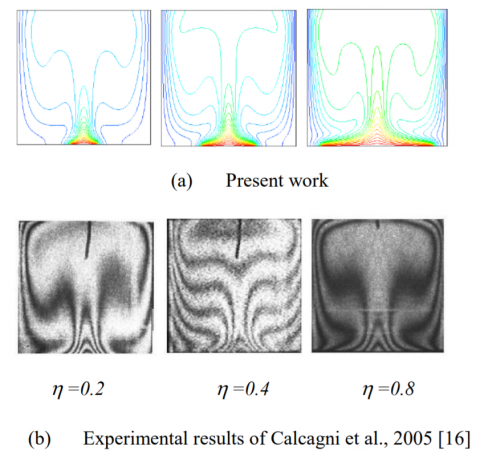Figure 2. Comparison of our results with those experimental data (Calcagni et al., 2005 ): Isothermal lines in the cavity for three cases: $\eta$ = 0.2 and Ra = 1.899×105 (left), $\eta$ = 0.4 and Ra = 1.86×105 (middle) and $\eta$ = 0.8 and Ra = 1.836×105 (right).

Table 1.  Flow Parameter: Umax, Vmax and Nuavg for different grid size (Gr = 109, Re = 105 and Ri = 0.1)

 Grid size Umax Vmax Nuavg 102×102 1,02 0,8566 8,3102 122×122 1,032 0,79 6,9043 142×142 1,048 0,7965 6,1899 162×162 1,092 0,779 5,4503

4. Results and Discussion

Currently, in ventilated enclosure in the presence of a heat source, the flow into the system is strongly depended to the Reynolds number (mixed convection). From a critical value of this parameters, the flow changes from laminar to turbulent regimes. The fluid is then animated random eddies. It is stirred in all directions and there is more stratified flow. To clarify the study of turbulent mixed convection in the presence of a heat source, we present below two geometric forms of the heat source: square and circular with different dimensions.

4.1 Square heat source

In the studied problem to retain the flow regime as turbulent we set the Grashof number at 109, the Reynolds number is based on the width of the inlet opening and the flow velocity of the inlet air, and takes different values ​​so that the Richardson number, indicating the importance of the type of natural or forced convection, takes the following values: Ri = 0.01, 0.05, 0.1, 1, 2, 5, 10, 20 and 30. Figures 3a-i represents the paths of some fluid particles for different Ri inside the cavity containing the square heat source.

## figure_3.png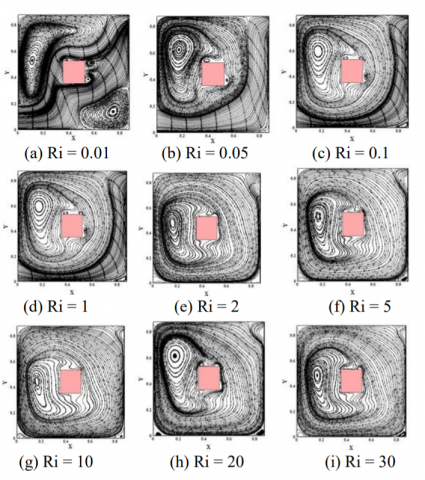Figure 3. Paths of some fluid particles in the cavity containing a square heat source, for different Richardson number (Ri = 0.01, 0.05, 0.1, 1, 2, 5, 10, 20 and 30).

From the analysis of the results of Figures 3a-i paths of some fluid particles in the cavity, we can note that the resulting mode is characterized by a vortex structure that changes with the increase of Ri. For Ri = 0.01, we can see the formation of two vortices (Fig. 3a): one, the largest, is in elongated form in the left. It rotates counter clockwise. And another, smaller one is to the right (lower right corner), and rotates clockwise. When the Ri is gradually increasing, the size of the vortex which rotates in the counter clockwise direction becomes greater; and therefore, the size of which rotates clockwise is reduced considerably. From Ri = 10, wherein the natural convection becomes predominant, the left vortex size becomes larger. Figures 4a-b, represent the profile of the dimensionless velocity component U according to Y (X = 0.5); and V according to X (Y = 0.5), for different Ri. We find that the maximum magnitude of velocity Umax and Vmax correspond to the value of Ri = 30; or the reduction of this latter induces the decrease in the velocity magnitude. Also, for Ri = 0.1 the change in the velocity field is very low in comparison with other values ​​of Ri. Figure 4-c shows the profiles of the dimensionless temperature at the middle of the cavity for different Ri. It’s clear that the increase of Ri increase the temperature at the neighboring of the two opening. It is notified that the profile is discontinued at the place of heat source (temperature is maximal, θ = 1).

Figures 5a-i, represent the isothermal line for different Ri. We find that the high temperatures are located in the near areas of the walls of the heat source that correspond to the thickness of the thermal layers that are largely influenced by Ri. Also, away from the hot walls, the temperature gradients are low. For low values ​​of Ri we see the presence of the thermal stratification in the right and upper walls of the heat source due to the effect of the air stream through the cavity to the outlet by increasing of Ri. The stratification in question of the right wall has disappeared, usually occurring in the upper wall of the heat source due to increased buoyancy forces. We note also that the thermal stratification begins to appear for large values ​​of Ri in both cases the predominant natural or forced convection:

• For the predominant forced convection, the thermal stratification occurs if  $0.05 \leq R i \leq 5$(Fig.5a-f).
• For the predominant natural convection, the thermal stratification occurs if  $20 \leq R i \leq 30$(Fig.5h-i).
• If we compare the Figs.5a-i, we can note that when Ri increases, the isothermal approach each other in the area near the hot walls of the heat source. In other words, the temperature gradient becomes higher near the heated walls. This last result on the isotherms is valid for two cases of predominant natural or forced convection.

## figure_4a.png(a)

## figure_4b.png(b)

## figure_4c.png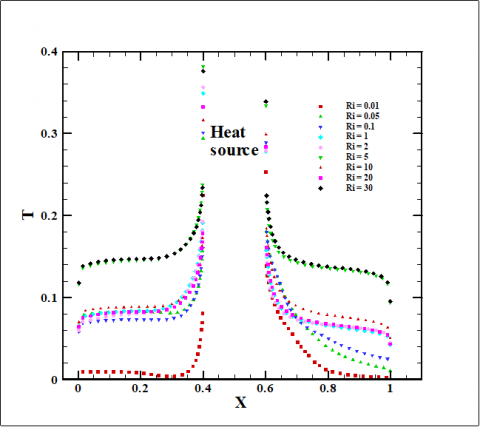(c)

Figure 4. Profiles of dimensionless velocity components (a) horizontal component, (b) vertical component, and (c) profile of dimensionless temperature according to the Richardson number at the middle of the cavity

## figure_5.png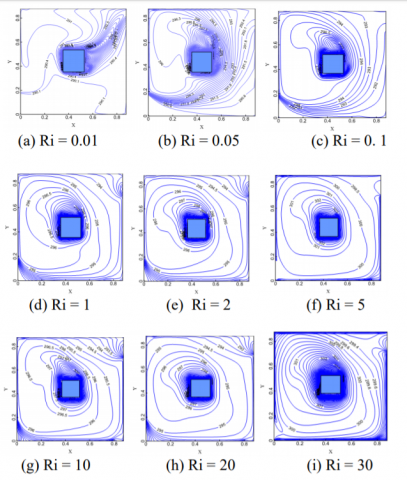Figure 5. Isothermal lines in the cavity containing a square heat source, according to Ri.

4.1.1 Effect of heat source dimensions (D)

Figures 6a-e show the paths of some fluid particles in the cavity containing a square heat source according to their dimensions (D) for Ri = 0.1. The dimension of the square heat source D is varying in according to the length of opening cavity h: D = 0.5h, h, 1.5h, 2h and 3h. We can see that for D = 3h, there is a large flow along the bottom wall which extends along the right vertical wall to the exit. A more decreases in heat source dimension D provide a low flow of the forced convection. For the case of D = 0.1h we see the great cell of natural convection. So, we conclude that the increase of the heat source dimension D provide the dominance of the forced convection. Therefore a good ventilation of the cavity.

## figure_6.pngFigure 6. Paths of some fluid particles in the cavity containing a square heat source according to the dimensions of square heat source (D), for Ri = 0.1.

4.1.2 Effect of dimension of inlet/outlet air flow

To study the influence of the variation of the inlet opening and the air outlet of the cavity (h) on the thermal and fluid dynamic parameters, it’s sound to choose arbitrary the values ​​of h depending to the side of the cavity: h = L /3, h = L /4 and h = L /10. Figures 7a-c, show the path of some fluid particles in the cavity for three preceding values ​​of h while Ri takes the following values ​​: 0.1, 5 and 30. For Ri = 0.1, where the forced convection is dominant. It can be see a large air jet form h = L/10 which tends to pass through the cavity to the outlet. For Ri = 5 (mixed convection) and for Ri = 30 (dominant natural convection). Therefore, the dimension of the inlet/outlet air flow can be changing the flow regimes when this dimension increased.

## figure_7a.png## figure_7b.pngFigure 7. Effect of varying of the opening of the inlet and outlet of the cavity (h), containing a square heat source, for (a) Ri = 0.1 (b) Ri = 5 and (c) Ri = 30

4.2 Circular heat source

To study the influence of heat source shape on the thermal and fluid dynamic parameters we have chosen a circular shape of the heat source of diameter “d”. For an objective comparison between the two sources of geometric shapes (square/circular), we keep the same parameters already studied for a square heat source such as: Prandtl number equal to 0.71, Grashof number is set to 109 and Richardson Number takes the following values ​​: 0.01, 0.05, 0.1, 1, 2, 5, 10, 20 and 30. The flow in the cavity is governed by the presence of a vortex air stream which passes through the cavity of the lower left corner to the upper right corner (Figs. 8a-i). For Ri = 0.01, there is a simple structure of the trajectories of fluid particles reflecting a pure and fast ventilation. Also, the formation of a great vortex playwright the circular source (see Fig.8a). For $0.01<\mathrm{Ri} \leq 1$, the formation of a single vortex, which occupies the entire cavity and increases with the increase of Ri, along with the disappearance of the air jet is noted. For $2 \leq \mathrm{Ri} \leq 10$, in this zone, it is noted the presence of the vortex air stream and which change with increasing Ri. For Ri = 2, we note the presence of three vortexes. For both the greatest, one occupies the entire cavity and the other smaller is located above the heat source near the hot wall. The two vortexes are rotating in the counter clockwise direction. These two together two cells which have the same direction of rotation. According to this movement, so the door cold air turbulence away nears the hot wall, and the other two are similar in size and are located one in the upper left corner and the other in the lower right corner. These two small vortexes rotate clockwise. When the Richardson number increases (Ri = 5) the size of the vortex which rotate counter clockwise increases, and accordingly the size of those which rotate clockwise decreases considerably. If we increase the Richardson number (Ri = 10), we see the appearance of the last two small vortex rotating clockwise. The existence of the air jet and the vortex unstable within this area indicates the development of the mixed convection (the effect of the forced or natural convection does not predominate). When the Richardson number (Ri = 20) wherein the natural convection is predominant, a large recirculation zone occupies the cavity.

The contours of the pressure around the circular heat source (Figs.9a-i) show that the decreasing of the drop pressure has been registered at the place of the dominating natural convection (blue area on Fig.9b, 9c, 9d, 9h and 9i). This in accordance with the velocity field indicated in the Figures 8a-i. So, that the pressure drop is a stronger constraint in the design of thermal systems rather than heat transfer. This results in good agreement with those of Madadi and Balaji 2008 .

## figure_8.pngFigure 8. Paths of some fluid particles in the cavity containing a circular heat source (D = 0.5h) according to Ri.

## figure_9.pngFigure 9. Pressure contours in the cavity containing a circular heat source (D = 0.5h) according to the Ri.

Figure 10a shows the dimensionless temperature profiles along Y = 0.5. The results are almost like those of the heat source square where we see values corresponding to the temperature of both left and right of the hot heat source. Thus, we see two weak values, the middle of the cavity which corresponds to the two ends of the enclosure. For the case of the circular source the following results are obtained: For low values ​​of Ri where the forced convection is dominant, there is a sudden drop in temperature. This is due to significant presence of the air in the area where the speed of passage of the particles is higher jet. This causes a significant drop in temperature. And for the other two areas of mixed convection and natural convection of predominantly the two lower values ​​corresponding to the two ends of the cavity are the same, because of the disappearance of the material flow and the presence of a large structure vortex occupying the entire cavity. The characteristics of turbulence are represented by the turbulent kinetic energy dissipation rate and turbulent viscosity (Figs. 10b-c). It is notified that the larger maximum of the turbulent kinetic energy values ​​and the rate of dissipation of turbulence Richardson numbers correspond to the following: Ri = 0.1, Ri = 1, Ri = 20 and Ri = 30, where the presence of flow is high (the air jet is composed of parallel flow lines see Figures 8a-i). The maximum values ​​of the turbulent kinetic energy correspond the following: Ri = 0.01, 0.05, 2, 5, 10 and where the air stream is reduced or eliminated. This result is due to the turbulence intensity that is measured by the turbulent kinetic energy dissipation rate and decreased by the presence of air jet lines of regular current. And consequently, a decrease in the turbulent kinetic energy should be noted. According to the turbulence model k-ε standard adopted in this study, the turbulent viscosity is defined by equation$\mu_{t}=\rho C_{\mu}\left(\frac{k^{2}}{\varepsilon}\right)$. For Ri where the air flow is apparent, we see that large values ​​are close to the air outlet in the upper right part of the cavity. This is due to the effect of blocking the flow through the upper horizontal wall which leads to secondary recirculation near the outlet. Therefore, the increase of turbulent kinetic energy. Variation there of influences the eddy viscosity for the two are proportional. By increasing Ri (Ri = 20 and Ri = 30), the maximal approach increasingly to the hot wall of the heat source, following the increase in temperature. This is justified by the increase in viscosity with increasing temperature for the gas; unlike liquids. Creating secondary movements in this case is due not only to the effect of locking, but also to the Coanda effect, which occurs when the speed is very high. Away from the hot wall, the turbulent viscosity is low especially for great Ri.

To study the influence of the dimensions of the circular heat source on the thermal and fluid dynamic parameters we chose arbitrary the values ​​of the following dimension: d = 0, 0.5h, h, 1.5h, and the selected Ri = 2. Figures 11a-d, represent the paths of some fluid particles in the cavity for different size of the circular heat source. For D = 0, the flow forms a large vortex structure which occupies the entire volume of the cavity. For D = 0.5h, we notice the presence of a large jet of air that tends to cross the cavity to the exit. Thus the formation of two vortexes: Firstly, the smallest is the lower right corner and rotates clockwise; and the second larger is above the heat source and to the left of the air stream. It rotates counter clockwise. For d = h, the small vortex disappears, and the air stream spreads along the two right and lower horizontal walls vertical to the exit. From D = 1.5h, the flow forms a large vortex structure that occupies the entire volume of the cavity.

## figure_10a.png(a)

## figure_10b.png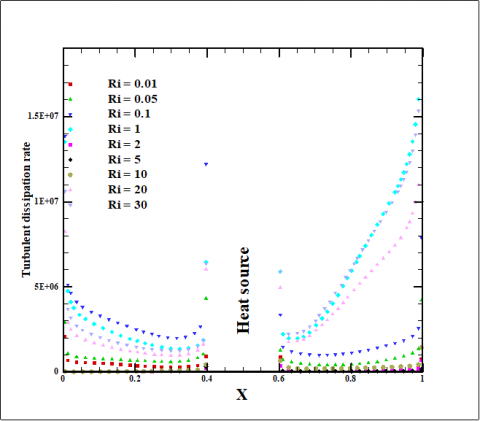(b)

## figure_10c.png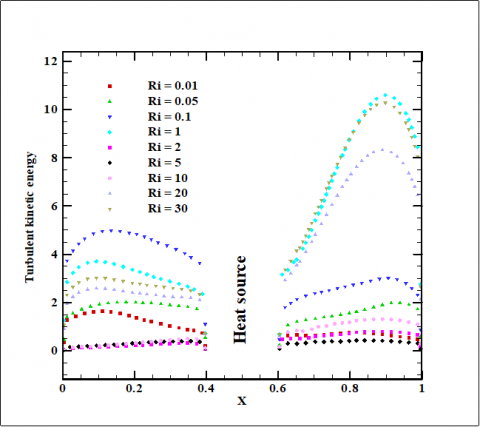(c)

Figure 10. Profiles of the dimensionless temperature T (a), turbulent dissipation rate (b), and turbulent kinetic energy (c), for different Ri

## figure_11.pngFigure 11. Path of some fluid particles in the cavity containing a circular heat source according to the size of circular heat source (Ri = 2)

4.3 Comparison between circular/square heat sources

The average heat transfer through on the hot wall was evaluated by the local Nusselt number. The Figure 12a shows the variation of the local Nusselt number on the upper horizontal wall of the square heat source according to the Richardson number Ri. This figure contains three distinct areas. For Ri = 0.1 to Ri = 1 where the forced convection is dominant the sudden drop in the local Nusselt is registered in the second middle of the enclosure x > 0.5. This can be explaining by the presence of the high ventilation in this part as we shown in Figures 6a-e, and significant fluctuations are remarkable where convection is mixed. From Ri = 10 the local Nusselt number decreases almost linearly with the increase of Ri where the natural convection is dominant.

The Figure 12b shows the local Nusselt number according to Richardson number of the upper semicircle of the circular heat source. According to the Table 2 below, we conclude that the heat transfer wall at the level of the active depends strongly on the geometry of the wall.

## figure_12a.png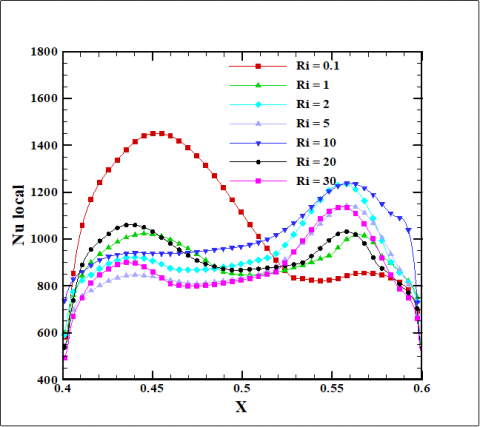(a)

## figure_12b.png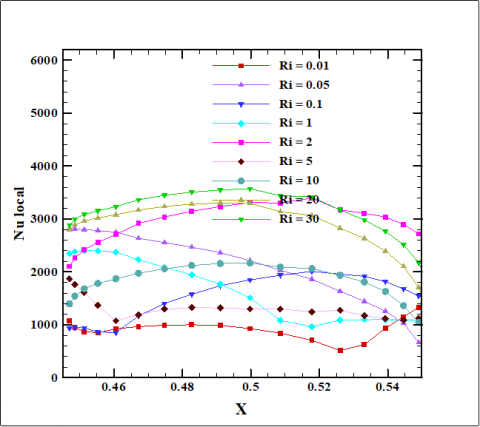(b)

Figure 12. Profiles of the local Nusselt number for both cases: square heat source (a), and circular heat source (b), according to the Richardson number Ri

Table 2. Average Nusselt Number for both sources circular/ square according to the Richardson number Ri

 Ri 0.01 0.05 0.1 1 5 10 20 30 Nuavg square source 982 1382 1451 1023 1143 1237 1061 1118 Nuavg circular source 1011 1890 1983 2399 1914 2164 3299 3560

If we compare the values ​​of the two cases of Nuavg of heat source for each value of Ri, we find that the circular form is better than square regarding the heat transfer rate. So, this leads to a circular shape better cooling of electronic components.

5. Conclusions

The turbulent mixed convection in a ventilated enclosure has been carried out. The influence of the geometry and dimensions of the heat source on the thermal and fluid dynamic parameters circular/square was studied. The analyses of the obtained results show that:

• The ranges of variation of the convection mode (forced, natural or mixed) according to the Richardson number Ri are different for two geometric shapes heat source square/circular and their dimensions.

- For the circular heat source:

$0.01<\mathrm{Ri} \leq 1$: forced convection is predominant.

$2<\mathrm{Ri} \leq 10$: mixed convection (The effect of forced or natural convection does not predominate).

$\mathrm{Ri} \geq 20$, natural convection is predominant.

- For the square heat source:

$0.01<\mathrm{Ri} \leq 1$: forced convection is predominant.

$2<\mathrm{Ri} \leq 10$: natural convection is predominant.

The range of the mixed convection between the two previous tracks is not well defined.

• Thermal and dynamic parameters of the fluid in the cavity are highly dependent to the dimension and the geometry of the heat source.
• A better cooling of the heat source is obtained for the circular shape.
• The flow structure and the heat transfer rate is highly dependent to the convective system and the size of the heat source.
Acknowledgment

This work was supported by the Research Team: Heat Transfer and Renewable Energy (HTRE) attached to the Mechanical Laboratory (LME), University of Laghouat that approved in 2009 under the number: 222 in 07/13/2009. The authors also gratifully acknowledge the helpful and suggestions of the reviewers, which have improved the presentation.

Nomenclature

D         Dimension/diameter of the heat source (m).

Gr       Grashof Number

g         Gravitational acceleration (m s-2)

K        Turbulent dissipation rate

h         Length of opening cavity

H        Dimensionless ratio.

L         Length of cavity

Nu       Nusselt number

p          Pressure (Pa)

P         Dimensionless pressure

Pr        Prandtl number

Ra       Rayleigh number

Re       Reynolds Number

Ri        Richardson Number

t          Temperature (K)

T         Dimensionless temperature

u, v     Velocity components (m s-1).

U, V    Dimensionless velocity components

x, y      Coordinates (m)

X, Y    Dimensionless coordinates

Greek symbols

α       Thermal diffusivity (m2 s-1)

βT      Thermal expansion coefficient (K-1)

μ       Dynamic viscosity (kg m-1 s-1)

ν        Kinematic viscosity (m2 s-1)

ρ       Density (kg. m-3)

$\eta$          Area ratio of source/bottom surface of enclosure.

Subscripts

Avg   average value

References

 G. Barakos and E. Mitsoulis, “Natural convection flow in square cavity revisited:   laminar and turbulent models with wall functions,” Int. J. Numerical Methods in Fluids, vol. 18, pp. 695-719, 1994. DOI: 10.1002/fld.1650180705.

 K.J. Hsieh and F.S. Lien, “Numerical modeling of buoyancy-driven turbulent flows in enclosures” International Journal of Heat and Fluid Flow, vol. 25 659–670, 2004. DOI: 10.1016/j.ijheatfluidflow.2003.11.023.

 M.T. Bhoite, G.S.V.L. Narasimham and M. V. Krishna Murthy, “Mixed convection in a shallow enclosure with a series of heat generating components,” International Journal of Thermal Sciences, vol. 44, pp. 121–135, 2005.  DOI: 10.1016/j.ijthermalsci.2004.07.003.

 S. Saha, G. Saha, M. Ali and Md.Q. Islam, “Combined free and forced convection inside a two-dimensional multiple ventilated rectangular enclosure,” Journal of Engineering and Applied Sciences, vol.1, No.3, 2006.

 Md.M. Rahman, M.A. Alim, S. Saha and M.K. Chowdhury, “Mixed convection in a vented square cavity with a heat conducting horizontal solid circular cylinder,” Journal of Architecture and Marine Engineering, vol. 2, pp. 37-46, 2008. DOI: /10.3329/jname.v5i2.2504.

 R. R. Madadi and C. Balaji, “Optimization of the location of multiple discrete heat sources in a ventilated cavity using artificial neural networks and micro genetic algorithm,” International Journal of Heat and Mass Transfer, vol. 51, pp. 2299–2312, 2008. DOI: 10.1016/j.ijheatmasstransfer.2007.08.033.

 S. Saha, M. N. Hasan and I. Ahmed Khan, “Double diffusive mixed convection heat transfer inside a vented square cavity,” Chemical Engineering Research Bulletin, vol. 13, pp. 17-24, 2009. DOI: 10.3329/cerb.v13i1.2512.

 S. Parvin and R. Nasrin, “Effects of Reynolds and Prandtl Number on mixed convection in an octagonal channel with a heat - generating hollow cylinder,” J. Sci. Res., vol. 4, no. 2, pp. 337-348, 2012. DOI: 10.3329/jsr.v4i2.8142.

 M. A. Sheremet and N. I. Shishkin, “Mathematical simulation of convective-radiative heat transfer in a ventilated rectangular cavity with consideration of internal mass transfer,” Journal of Engineering Physics and Thermophysics, vol. 85, no. 4, 2012. DOI: 10.1007/s10891-012-0720-z.

 A. Andreozzia, N. Biancoa, O. Mancab and V. Nasoa, “Turbulent mixed convection in a uniformly heated vertical channel with an assisting moving surface,” International Journal of Thermal Sciences, vol. 71, pp. 20-31, 2013.  DOI: 10.1016/j.ijthermalsci.2013.04.001.

 K. Bouaraour, A. Belaid, “Effect of Inlet Width on Turbulent Flow in a Vented Cavity with an Isothermal Vertical Wall,” International Journal of Sciences:  Basic and Applied Research, vol. 24, no. 3, pp.14 -26, 2015.

 N. A. Rodriguez, J. F. Hinojosa and J. Xaman, “Comparative study between experimental data and numerical results of turbulent mixed convection in a ventilated cavity,” J. Heat Transfer, vol. 137, no. 5, 2015. DOI: 10.1115/1.4029646.

 S. K. Ajmera , A.N. Mathur, “Combined free and forced convection in an enclosure with different ventilation arrangements,” Procedia Engineering, vol. 127, pp. 1173-1180, 2015. DOI: 10.1016/j.proeng.2015.11.456.

 Atia Aissa, Bouabdallah Said, Teggar Mohamed and Benchatti Ahmed, “Numerical Study of mixed convection in cylindrical Czochralski configuration for crystal growth of silicon” International Journal of Heat and Technology, vol. 33, no. 1, pp. 39-46, 2015. DOI: 10.18280/ijht.330106.

 Badia Ghernaout, Said Bouabdallah, Mohamed Teggar and Hamza Benniche, “Double diffusive natural convection in binary mixture under the effect of external magnetic field: steady and oscillatory state,” International Journal of Heat and Technology. vol. 33, no. 4, pp. 11-18, 2015.  DOI: 10.18280/ijht.330402.

 B. Calcagni, F. Marsili and M. Paroncini, “Natural convective heat transfer in square enclosures heated from below,” Applied Thermal Engineering, vol. 25, pp. 2522–2531, 2005.  DOI: 10.1016/j.applthermaleng.2004.11.032.